Courses

# Reversible Reactions And Equilibrium - Chemical Kinetics Chemistry Notes | EduRev

## Chemistry : Reversible Reactions And Equilibrium - Chemical Kinetics Chemistry Notes | EduRev

The document Reversible Reactions And Equilibrium - Chemical Kinetics Chemistry Notes | EduRev is a part of the Chemistry Course Physical Chemistry.
All you need of Chemistry at this link: Chemistry

Reversible reactions and Equilibrium

Consider the following reaction in which the forward reaction is first order in A, and the back reaction is first order in B :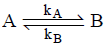The forward and back rate constant are kA & kB. Then rate law are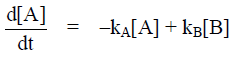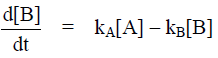Only reactant is present at t = 0 and the concentration of reactant and product for t > 0 must be equal to the initial concentration of reactant. [A]0 = [A] + [B]
then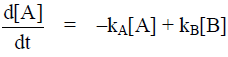= –kA[A] + kB[B]
= –kA[A] + kB([A]– [A])
= –[A] (kA + kB) + kB[A]0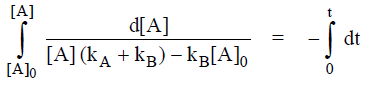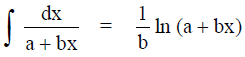Using this relationship we get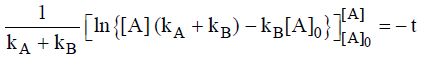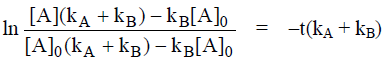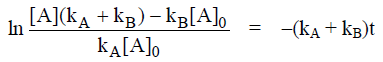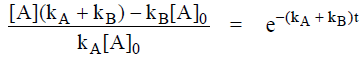[A] (kA + kB) – kB[A]0 = k[A]0 e-( kA+ kB)t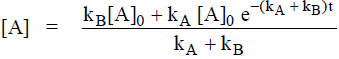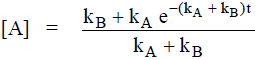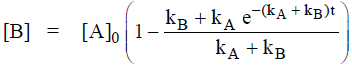Then

As t → ∝, the concentration reach their equilibrium values then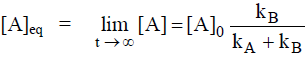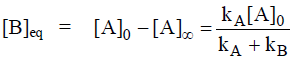It follows that the equilibrium constant of the reaction is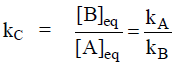i.e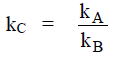kC is equilibrium constant in terms of concentration.
At equilibrium, the forward and reverse rates must be same so,

KA[A]eq = kB[B]eq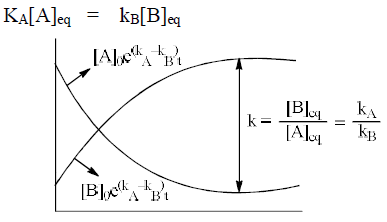Problem.  Using the following equation
A → C

mechanism,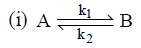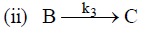(a)  Find rate of reaction ?
(b)  Find rate of reaction when (i) is fast.
Sol. (a) From the rate law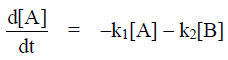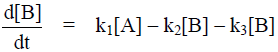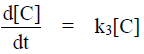[B] is intermediate then we apply SSA then we get

0 = k1[A] – k2[B] – k3[B]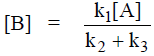then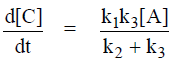(b) When (i) is fast then

k1[A] = k2[B]
i.e.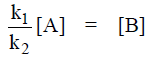&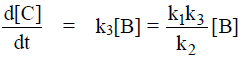Problem.  Using the following equation
2NO2 + F2 → 2NO2F
Mechanism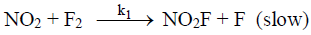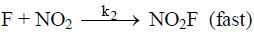Find the rate of reaction.
Sol.
From the rate law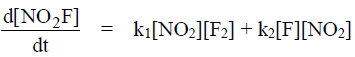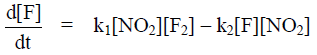= 0 (F is intermediate)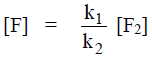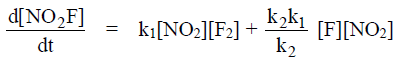= 2k1[F2][NO2]

Offer running on EduRev: Apply code STAYHOME200 to get INR 200 off on our premium plan EduRev Infinity!

## Physical Chemistry

66 videos|94 docs|32 tests

,

,

,

,

,

,

,

,

,

,

,

,

,

,

,

,

,

,

,

,

,

;